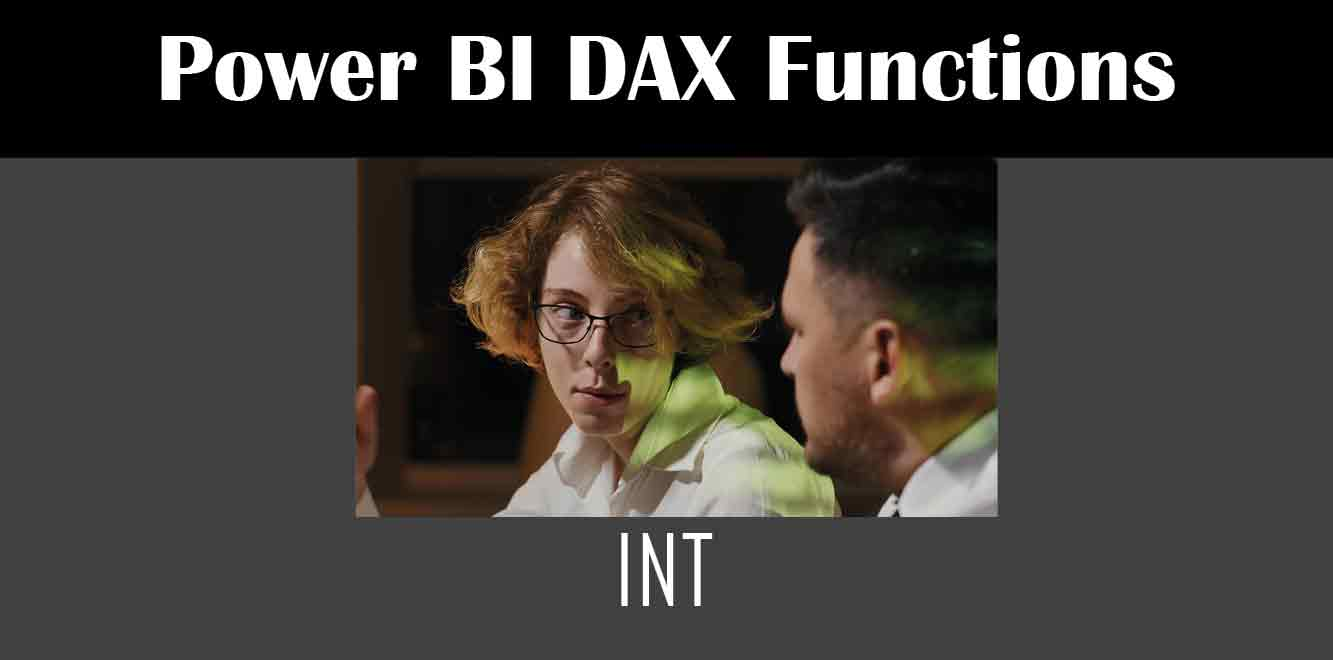# INT

## Y## Syntax of the INT Function

The syntax of the INT function is as follows:

INT(❰number❱)

``` Here, `❰number❱` is the number that you want to round down to the nearest integer. Usage of the INT Function The INT function can be used in a variety of scenarios. Let's take a look at a few examples: Example 1 Suppose you have a Sales table in your Power BI report with a column named `Revenue`. You want to calculate the total revenue for each product category, rounded down to the nearest whole number. You can achieve this using the following DAX formula: ```

Total Revenue = SUMX(‘Sales’, INT(‘Sales'[Revenue]))

``` Here, `SUMX` calculates the sum of the values returned by the `INT` function for each row in the Sales table. Example 2 Suppose you have a Customer table in your Power BI report with a column named `Age`. You want to calculate the average age of all customers, rounded down to the nearest whole number. You can achieve this using the following DAX formula: ```

Average Age = INT(AVERAGE(‘Customer'[Age]))

``` Here, `AVERAGE` calculates the average of the values in the `Age` column, and the `INT` function rounds the result down to the nearest whole number. Example 3 Suppose you have a table named `Inventory` in your Power BI report with a column named `Units in Stock`. You want to calculate the total number of units in stock that are in multiples of 10. You can achieve this using the following DAX formula: ```

Total Units in Stock = SUMX(‘Inventory’, INT(‘Inventory'[Units in Stock]/10)*10)

``` Here, the `INT` function rounds the value in the `Units in Stock` column down to the nearest multiple of 10, and then multiplies it by 10 to get the final value. The INT function in DAX is a powerful tool that can be used to round off numbers to the nearest whole number. It is a simple and easy-to-use function that can be used in a variety of scenarios. We hope this article has helped you understand the syntax and usage of the INT function in Power BI. Power BI DAX Training Courses by G Com Solutions (0800 998 9248)Power BI DAX Intensive Training Course £1,050.00 – £26,550.00 Select optionsContinue Loading DonePower BI DAX Introduction £395.00 – £9,750.00 Select optionsContinue Loading DonePower BI DAX Intermediate £395.00 – £9,750.00 Select optionsContinue Loading DonePower BI DAX Advanced £395.00 – £9,750.00 Select optionsContinue Loading Done Upcoming Courses Contact Us Subject Your Name (required) Company/Organisation Email (required) Telephone Training Course(s) Power BI Intensive TrainingPower BI introduction Power BI IntermediatePower BI AdvancedDAXPower Query MPower BI CertificationPower BI AdministrationPower PlatformPower AutomatePower AppsOTHER Your Message Upload Example Document(s) (Zip multiple files) ```
``` ```
``` ```
``` ```
``` ```
``` ```